JEE  >  VITEEE PCME Mock Test - 14

# VITEEE PCME Mock Test - 14

Test Description

## 125 Questions MCQ Test VITEEE: Subject Wise and Full Length MOCK Tests | VITEEE PCME Mock Test - 14

VITEEE PCME Mock Test - 14 for JEE 2023 is part of VITEEE: Subject Wise and Full Length MOCK Tests preparation. The VITEEE PCME Mock Test - 14 questions and answers have been prepared according to the JEE exam syllabus.The VITEEE PCME Mock Test - 14 MCQs are made for JEE 2023 Exam. Find important definitions, questions, notes, meanings, examples, exercises, MCQs and online tests for VITEEE PCME Mock Test - 14 below.
Solutions of VITEEE PCME Mock Test - 14 questions in English are available as part of our VITEEE: Subject Wise and Full Length MOCK Tests for JEE & VITEEE PCME Mock Test - 14 solutions in Hindi for VITEEE: Subject Wise and Full Length MOCK Tests course. Download more important topics, notes, lectures and mock test series for JEE Exam by signing up for free. Attempt VITEEE PCME Mock Test - 14 | 125 questions in 150 minutes | Mock test for JEE preparation | Free important questions MCQ to study VITEEE: Subject Wise and Full Length MOCK Tests for JEE Exam | Download free PDF with solutions
 1 Crore+ students have signed up on EduRev. Have you?
VITEEE PCME Mock Test - 14 - Question 1

### When the function f(x) = sin 2x(1 + cos 2x) has a maximum, one value of x is equal to

VITEEE PCME Mock Test - 14 - Question 2

### The area bounded by the curve y = x3 , x-axis and two ordinates x = 1 to x = 2 is equal to

VITEEE PCME Mock Test - 14 - Question 3

### The roots of the equation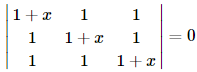Detailed Solution for VITEEE PCME Mock Test - 14 - Question 3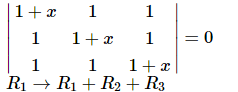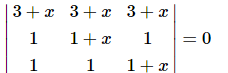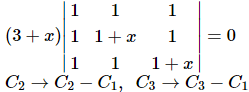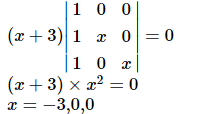VITEEE PCME Mock Test - 14 - Question 4

If A, B, C are represented by 3 + 4i, 5 - 2i, -1 + 16i respectively, then A, B, C are

VITEEE PCME Mock Test - 14 - Question 5

If α , β are two different complex numbers such that | α | = 1, | β | = 1, then the expression | β − α /1 − α β | equals

VITEEE PCME Mock Test - 14 - Question 6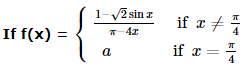is continuous at π/4 , then a =

VITEEE PCME Mock Test - 14 - Question 7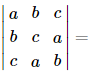VITEEE PCME Mock Test - 14 - Question 8

Consider f (x) = x2 /| x | , x ≠ 0, f(x) = 0, x = 0

VITEEE PCME Mock Test - 14 - Question 9

The area bounded by the curve y = x2 - 4x, x-axis and line x = 2 is

Detailed Solution for VITEEE PCME Mock Test - 14 - Question 9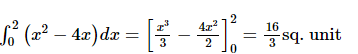VITEEE PCME Mock Test - 14 - Question 10

Find the degree of (y2)2 − √y1 = y3

VITEEE PCME Mock Test - 14 - Question 11

The solution of differential equation (xdy/dx)=y+x2 is

VITEEE PCME Mock Test - 14 - Question 12

The degree and order of the differential equation of the family of all parabolas whose axis is x-axis are respectively

VITEEE PCME Mock Test - 14 - Question 13

The differential equation of the family of curves y2=4a (x+a) is

VITEEE PCME Mock Test - 14 - Question 14

If x=a(t-sint) and y=a(1-cost), (dy/dx)=

VITEEE PCME Mock Test - 14 - Question 15

If f(x) is an odd periodic function with period 2, then f(4) equals :

VITEEE PCME Mock Test - 14 - Question 16

∫tan-1x dx =

VITEEE PCME Mock Test - 14 - Question 17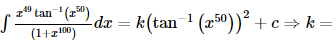Detailed Solution for VITEEE PCME Mock Test - 14 - Question 17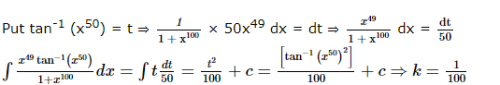VITEEE PCME Mock Test - 14 - Question 18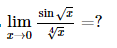VITEEE PCME Mock Test - 14 - Question 19

If tan-1x + tan-1y + tan-1z = π, then x+y+z is equal to

VITEEE PCME Mock Test - 14 - Question 20

A function f(x) is defined as f (x) = [ 1 − x2 ] , − 1 ≤ x ≤ 1 , where [x] denotes the greatest integer not exceeding x. The function f(x) is discontinuous at x = 0 because

VITEEE PCME Mock Test - 14 - Question 21

The sentence "____ if and only if x + x = 3x" is TRUE.

Detailed Solution for VITEEE PCME Mock Test - 14 - Question 21

"if and only if" is TRUE when both conditions are the SAME truth value. Since "x + x = 3x" is false, the first condition must also be false. 2x - x = 2x is false.

VITEEE PCME Mock Test - 14 - Question 22

A and B are square matrices of the same order. Which is correct?

VITEEE PCME Mock Test - 14 - Question 23

If A and B are two matrices such that AB=B and BA=A, then A2+B2=

VITEEE PCME Mock Test - 14 - Question 24

If nPr=840, nCr=35, then n=

VITEEE PCME Mock Test - 14 - Question 25

A biased coin with probability p,0<p<1 of heads is tossed until a head appears for the first time. If the probability that the number of tosses required is even, is 2/5, then p equals

VITEEE PCME Mock Test - 14 - Question 26

In two events P(A∪B) = 5/6, P(A) = 5/6, P(B) = 2/3 then A and B are

VITEEE PCME Mock Test - 14 - Question 27

In four schools B₁, B₂, B₃, B₄ the percentage of girls students is 12, 20, 13, 17 respectively. From a school selected at random, one student is picked up at random and it is found that the student is a girl. The probability that the school selected is B₂, is

VITEEE PCME Mock Test - 14 - Question 28

If the side of a triangle are 13, 14, 15, then the radius of the incircle is

VITEEE PCME Mock Test - 14 - Question 29

The fourth ,seventh and tenth of a G.P. are p,q,r respectively then

VITEEE PCME Mock Test - 14 - Question 30

Two numbers within the brackets denote the ranks of 10 students of a class in two subjects - (1, 10), (2, 9), (3, 8), (4, 7), (5, 6), (6, 5), (7, 4), (8, 3), (9, 2), (10, 1), then rank correlation coefficient is

VITEEE PCME Mock Test - 14 - Question 31

The mean of 5 observations is 4.4 and their variance is 8.24. If three observations are 1, 2 and 6, the other two observations are

VITEEE PCME Mock Test - 14 - Question 32

The one roots of the equation 2x5-14x4+31x3-64x2+19x+130=0 is

VITEEE PCME Mock Test - 14 - Question 33

The equation of the sphere concentric with the sphere x2+y2+z2-4x-6y-8z=0 and which passes thro' (0.1,0) is

VITEEE PCME Mock Test - 14 - Question 34

If α , β , γ are the roots of the equation x3 − 6 x2 + 11 x + 6 = 0, then Σ α2 β + Σ α β2 =

VITEEE PCME Mock Test - 14 - Question 35

The locus of the point of intersection of tangents to the circle x = a cos θ, y = a sin θ at the points, whose parametric angles differ by π/2 is

VITEEE PCME Mock Test - 14 - Question 36

3x (x - 2) + 3y (y + 1) = 4 is

VITEEE PCME Mock Test - 14 - Question 37

In three dimensional space, the equation 3y+4z=0 represents

VITEEE PCME Mock Test - 14 - Question 38

If vectors 2i+j+k and i-4j+λk are perpendicular, then λ=

VITEEE PCME Mock Test - 14 - Question 39

If the solution for θ of cospθ + cosqθ = 0, p > 0, q > 0 are in A.P., then the numerically smallest common difference of A.P. is

Detailed Solution for VITEEE PCME Mock Test - 14 - Question 39

Given cospθ = - cosqθ = cos (π + qθ)
⇒ pθ = 2nπ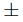(π + qθ), n ∈ I
⇒ θ = (2n + 1)π/p - q or (2n - 1)π/p + q , n ∈ I
Angle θ = (2n + 1)π p - q given us an AP with common
difference 2π/p - q and θ = (2n - 1)π/p + q gives also an AP with common difference 2π/p + q
Then, certainly, 2π/p + q < | 2π/p - q |
∴ The smallest common difference is 2π/p + q

VITEEE PCME Mock Test - 14 - Question 40

The projection of the vector î - 2 ĵ + k̂ on the vector 4 î - 4 ĵ + 7 k̂ is

VITEEE PCME Mock Test - 14 - Question 41

Sodium has body centred packing. Distance between two nearest atoms is 3.7 Å . The lattice parameter is:

VITEEE PCME Mock Test - 14 - Question 42

For series LCR circuit, wrong statement is

VITEEE PCME Mock Test - 14 - Question 43

The closest distance of approach of an α - particle travelling with a velocity 'V' to a certain nucleus is 'x'. The distance of closest approach of α - particle travelling with a velocity 3V to the same nucleus is

VITEEE PCME Mock Test - 14 - Question 44

A parallel plate condenser with oil between the plates (dielectric constant of oil K = 2) has a capacitance C. If the oil is removed, then capacitance of the capacitors becomes

Detailed Solution for VITEEE PCME Mock Test - 14 - Question 44

On removing the oil, the capacitance of the capacitor will become.
C ′ = C/K = C/2

VITEEE PCME Mock Test - 14 - Question 45

A steady current of 1.5 A flows through a copper voltameter for 10 min. If the electrochemical equivalent of copper is 30 x 10-5 g C-1 , the mass of copper deposited on the electrode will be

VITEEE PCME Mock Test - 14 - Question 46

A laser beam is used for carrying out surgery because it

Detailed Solution for VITEEE PCME Mock Test - 14 - Question 46

As the laser beam is highly monochromatic, directional and coherent, it can be sharply focussed.
Therefore it is used for carrying out surgery

VITEEE PCME Mock Test - 14 - Question 47

EMF is most closely related to

VITEEE PCME Mock Test - 14 - Question 48

Three resistors are connected to form the sides of a triangle ABC, the resistance of the sides AB, BC and CA are 40 ohms, 60 ohms and 100 ohms respectively. The effective resistance between the points A and B in ohms will be

VITEEE PCME Mock Test - 14 - Question 49

Taking the internal resistance of the battery as negligible, the steady state current in the 2 Ω resistor shown in the figure will be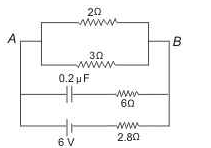VITEEE PCME Mock Test - 14 - Question 50

If two identical heaters of 220 V, 1000 W are placed parallel to each other across 220 V lines, then the combined power of the heaters is

VITEEE PCME Mock Test - 14 - Question 51

In a D.C. motor induced e.m.f will be maximum

VITEEE PCME Mock Test - 14 - Question 52

The approximated wavelength of a photon of energy 2.48eV is

VITEEE PCME Mock Test - 14 - Question 53

A 5 cm long solenoid having 10 cm resistance and 5 mH inductance is joined to a 10 V battery. At steady state, current through solenoid is

Detailed Solution for VITEEE PCME Mock Test - 14 - Question 53

At steady state, inductance is ineffective
I = V/R = 10/10 = 1A

VITEEE PCME Mock Test - 14 - Question 54

When the current in a coil is changed from 10 A in one direction to 10 A in opposite direction in 0.5 s, an e.m.f. of 1 V is induced in it. Self-inductance of the coil is

VITEEE PCME Mock Test - 14 - Question 55

Out of the folowing statement which is NOT true?

VITEEE PCME Mock Test - 14 - Question 56

If the electric flux entering and leaving an enclosed surface respectively is φ₁ and φ₂, the electric charge inside the surface will be

VITEEE PCME Mock Test - 14 - Question 57

Two equal charges q of opposite sign separated by a distance 2a constitute an electric dipole of dipole moment p. If P is a point at a distance r from the centre of the dipole and the line joining centre to this point makes an angle θ with the axis of the dipole, then potential at the point P is given by

VITEEE PCME Mock Test - 14 - Question 58

Two equal charges, q each are placed at a distance of 2a, and a third charge -2q is placed at the mid point. The potential energy of the system is

VITEEE PCME Mock Test - 14 - Question 59

The capacitance of a parallel plate capacitor becomes 4/3 times its original value if a dielectric slab of thickness t = d/2 is inserted between the plates (where d is separation between the plates). The dielectric constant of the slab is

VITEEE PCME Mock Test - 14 - Question 60

If an unchanged capacitor is charged by connecting it to a battery, then the amount of energy lost as heat is

VITEEE PCME Mock Test - 14 - Question 61

The inward and outward electric flux of a closed surface are 8x10C and 4x10C respectively. The net charge inside the closed surface is

VITEEE PCME Mock Test - 14 - Question 62

A 10 A ammeter has a resistance of 0.09 Ω. What resistance of the shunt will enable it to read up to 100 A?

VITEEE PCME Mock Test - 14 - Question 63

The first atomic reactor was constructed by

VITEEE PCME Mock Test - 14 - Question 64

The half life period of radio - active isotope is 5 minutes. The fraction of isotope, that will be remaining after 30 minutes is

VITEEE PCME Mock Test - 14 - Question 65

The number of beta particles emitted by a radioactive substance is twice the number of alpha particles emitted by it. The resulting daughter is an:

VITEEE PCME Mock Test - 14 - Question 66

When a metallic surface is illuminated by a monochromatic light of wavelength λ, then the potential difference required to stop the ejection of electrons is 3V₀. When the same surface is illuminated by the light of wavelength 2λ, then the potential difference required to stop the ejection of electrons is V₀. Then for photoelectric effect, the threshold wavelength for the metal surface will be

VITEEE PCME Mock Test - 14 - Question 67

The work function of a metal is 2.8 eV. Its threshold wavelength will be

VITEEE PCME Mock Test - 14 - Question 68

A telescope consists of two thin lenses of focal lengths 0.3 m and 3 cm respectively. It is focused on moon which subtends an angle of 0.50 at the objective. Then, the angle subtended at the eye by the final image will be

VITEEE PCME Mock Test - 14 - Question 69

Ultra-violet rays are detected by

VITEEE PCME Mock Test - 14 - Question 70

In the middle of the depletion layer of a reverse-biased p-n junction, the

VITEEE PCME Mock Test - 14 - Question 71

The method of connecting the negative pole of battery to p - material and positive pole to n - material of a p - n junction is called

VITEEE PCME Mock Test - 14 - Question 72

Rectifier is used to convert

VITEEE PCME Mock Test - 14 - Question 73

The band gap in Germanium and silicon in eV respectively is

VITEEE PCME Mock Test - 14 - Question 74

When germanium atoms is doped with equal no. of antimony atoms, we get a/an

VITEEE PCME Mock Test - 14 - Question 75

Suitable impurities are added to a semiconductor depending on its use. This is done to

VITEEE PCME Mock Test - 14 - Question 76

At room temperature, a p-type semiconductor has

VITEEE PCME Mock Test - 14 - Question 77

In interference pattern, the energy is

VITEEE PCME Mock Test - 14 - Question 78

Young's double slit experiment gives interference fringes of width 0.3mm. A thin glass plate made of material of refractive index 1.5 is kept in the path of light from one of the slits, then the fringe width becomes

VITEEE PCME Mock Test - 14 - Question 79

Which one of the following properties of light does not support wave theory of light?

VITEEE PCME Mock Test - 14 - Question 80

If ratio of amplitude of two waves is 4:3, then ratio of maximum and minimum intensity is

VITEEE PCME Mock Test - 14 - Question 81

Ethyl alcohol can be used for preparation of which of the following?

VITEEE PCME Mock Test - 14 - Question 82

Aldol condensation will not be observed in

VITEEE PCME Mock Test - 14 - Question 83

The base-catalysed aldol condensation will not occur with

VITEEE PCME Mock Test - 14 - Question 84

Which of the following reaction cannot be used for the conversion RCO → RCH₂

VITEEE PCME Mock Test - 14 - Question 85

Tetrabromomethane on treatment with Zn gives

VITEEE PCME Mock Test - 14 - Question 86

The product formed by the action of chlorine on ethene in saturated solution of KBr is/are

VITEEE PCME Mock Test - 14 - Question 87

In the following reaction, which choice has value twice that of the equivalent mass of the oxidising agent SO₂ + H₂O → 3S + 2H₂O

VITEEE PCME Mock Test - 14 - Question 88

Which species represents the electrophile in aromatic nitration?

VITEEE PCME Mock Test - 14 - Question 89

Lipids are

VITEEE PCME Mock Test - 14 - Question 90

Ethyl alcohol reacts with acetyl chloride to form

VITEEE PCME Mock Test - 14 - Question 91

Chargaff's rule states that in an organism

VITEEE PCME Mock Test - 14 - Question 92

A certain compound gives negative test with ninhydrin and positive test with Benedict's solution. The compound is

VITEEE PCME Mock Test - 14 - Question 93

Rancidity of butter is due to the formation of

VITEEE PCME Mock Test - 14 - Question 94

From the following values of dissociation constants of four acids which value represents the strongest acid ?

VITEEE PCME Mock Test - 14 - Question 95

The chemical equilibrium of a reversible reaction is not influenced by

VITEEE PCME Mock Test - 14 - Question 96

In the case of gaseous homogeneous reaction,the active mass of the reactant is obtained by the expression

VITEEE PCME Mock Test - 14 - Question 97

The internal energy of a subtance does not depend upon

VITEEE PCME Mock Test - 14 - Question 98

A compound with molecular formula C₇H₁₆ shows optical isomerism , the compound will be

VITEEE PCME Mock Test - 14 - Question 99

Which of these is a hypnotic?

VITEEE PCME Mock Test - 14 - Question 100

The heat of formation of the compound in the following reaction is H₂(g) + Cl₂(g) → 2HCl(g) + 44 kcal

VITEEE PCME Mock Test - 14 - Question 101

Calculate Δ H in KJ for the following reaction C(s) + O2 g → CO2 g Given that
H2O g + C g → CO g + H2  g , Δ H = + 131 k J
CO g + 1/2 O2  g → CO2  g , Δ H = − 282 k J
H2g + 1/2 O2 g → H2O g , Δ H = − 242 k J

VITEEE PCME Mock Test - 14 - Question 102

The complex CoCl₃.3NH₃ ionises to give

VITEEE PCME Mock Test - 14 - Question 103

The oxidation state of Cr in [ Cr (NH3)4 Cl 2 ] + is :

VITEEE PCME Mock Test - 14 - Question 104

Which of the following is produced by reaction of RCN in sodium and alcohol ?

VITEEE PCME Mock Test - 14 - Question 105

Among the properties (a) reducing (b) oxidising (c) complexing, the set of properties shown by CN⁻ ion towards metal species is

VITEEE PCME Mock Test - 14 - Question 106

Calculate the amount of change flowing in 2 minute in a wire of resistance 10 Ω when a potential difference of 20 V is applied

VITEEE PCME Mock Test - 14 - Question 107

Diazo coupling is useful to prepare

VITEEE PCME Mock Test - 14 - Question 108

On heating quick lime with coke in an electric furnace, we get

VITEEE PCME Mock Test - 14 - Question 109

Diethyl ether can be decomposed by

VITEEE PCME Mock Test - 14 - Question 110

The standard reduction potential at 298 K for the following half cell reaction are given below
Zn2+ + 2e-  → Zn E0 = − 0.762 V
Cr+3 + 3 e- → Cr E0  = − 0.740 V
2 H+ + 2 e → HE0  = − 0.0 V
Fe+3  + e-  → Fe+2  E0  = − 0.770 V
Which one is the strongest reducing agent?

VITEEE PCME Mock Test - 14 - Question 111

VITEEE PCME Mock Test - 14 - Question 112

The largest bond angle is in

VITEEE PCME Mock Test - 14 - Question 113

If the pressure and absolute temperatre of 2 litres of CO₂ are doubles, the volume of CO₂ would become

VITEEE PCME Mock Test - 14 - Question 114

Which one of the following is pyrophosphoric acid?

VITEEE PCME Mock Test - 14 - Question 115

Which substance is serving as a reducing agent in the following reaction? 14H⁺ + Cr₂O₇2- + 3Ni → 2Cr+3 + 7H₂O + 3Ni+2

VITEEE PCME Mock Test - 14 - Question 116

Following compounds are respectively ... geometrical isomers

VITEEE PCME Mock Test - 14 - Question 117

A face-centred cubic element has a edge length of 400 pm. What is its density? At. weight of element= 60)

VITEEE PCME Mock Test - 14 - Question 118

VITEEE PCME Mock Test - 14 - Question 119

Colourless solutions of the following four salts are placed separately in four different test tubes and a strip of copper is dipped in each one of these. Which solution will turn blue ?

VITEEE PCME Mock Test - 14 - Question 120

Highest oxidation state of transition element is

VITEEE PCME Mock Test - 14 - Question 121

Find the antonym of CORPULENT

VITEEE PCME Mock Test - 14 - Question 122

Fill in the blank with appropriate word.
You will have to face some practical problems when you start.........this plan.

VITEEE PCME Mock Test - 14 - Question 123

Find the Synonym of LANGUISHING

VITEEE PCME Mock Test - 14 - Question 124

Choose the alternative which best expresses the meaning of the idiom/phrase.

To be under a cloud

VITEEE PCME Mock Test - 14 - Question 125

Improve the sentence by choosing best alternative for capitalised part of the sentence.

He has very good command ON English.

## VITEEE: Subject Wise and Full Length MOCK Tests

1 videos|3 docs|73 tests
 Use Code STAYHOME200 and get INR 200 additional OFF Use Coupon Code
Information about VITEEE PCME Mock Test - 14 Page
In this test you can find the Exam questions for VITEEE PCME Mock Test - 14 solved & explained in the simplest way possible. Besides giving Questions and answers for VITEEE PCME Mock Test - 14, EduRev gives you an ample number of Online tests for practice

## VITEEE: Subject Wise and Full Length MOCK Tests

1 videos|3 docs|73 tests